# Programmatically creating radiating rows of dots

I want to create a speaker hole pattern like this: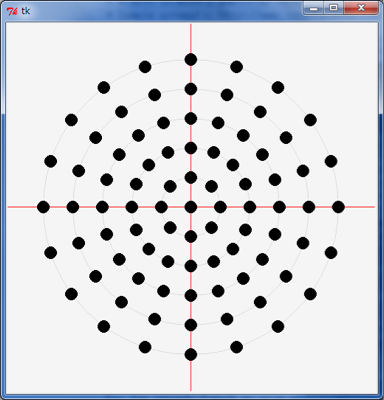But I’m not sure where to start. Can this be achieved without laborious positioning in Illustrator or similar software?

Thank you for visiting the Q&A section on Magenaut. Please note that all the answers may not help you solve the issue immediately. So please treat them as advisements. If you found the post helpful (or not), leave a comment & I’ll get back to you as soon as possible.

### Method 1

You dont actually specify whether or not the image is something you have generated yourself in TK, have at hand or not. If you already have this code then you can export the TK applications canvas as EPS and open it in illustrator. All you need to do is call `canvas.postscript()`.

#### If you want to use TK

Simple sample in python 2:

```#!/usr/bin/python
# -*- coding: utf-8 -*-

from Tkinter import *
import math

def circle(c, x, y, r=10):
return c.create_oval(x-r, y-r, x+r, y+r, width=0, fill="black")

def draw_circles(c, num, r):
step = (2.0*math.pi)/float(num)
for i in range(num):
x = 400 + r * math.sin(i*step)
y = 400 + r * math.cos(i*step)
circle(c, x, y)

main_window = Tk()
main_window.title('Pattern to EPS')
canvas = Canvas(main_window,
width=800, height=800,
bg = 'white')

circle(canvas, 400, 400)
for i in range(1, 6):
draw_circles(canvas, i*8, i*60)

canvas.pack()

# next line generates a eps file
canvas.postscript(file = "pattern.eps",  width=800, height=800 )

# uncomment next line if you want to see the tk window
# main_window.mainloop()```

This results in a file named `"patten.eps"`.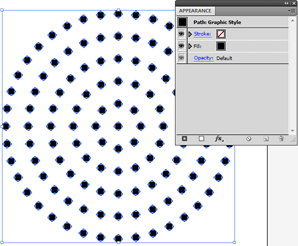Image 1: Opening the generated EPS in illustrator.

You can do this in extendScript, SVG or directly by writing the EPS program all of which are easy to do (see appendix below for some examples). See following posts for resources:

PS: I dont know if its worth scripting as it takes about 3 minutes to draw them in with help of rotate tool and Ctrl+D

#### Parametric blend in Illustrator

1. Draw a circle.
2. duplicate it.
3. blend the circles
4. draw another circle that represents the spine, cut it in one point
5. Select both blend and circle and do Object → Blend → Replace spine
6. adjust the number of spheres with Object → Blend → Blend Options… minus one object.
7. Copy and adjust spne circles size and options. done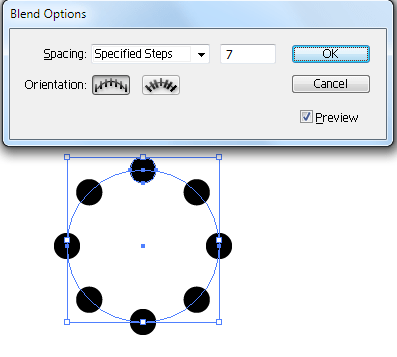Image 2: One ring with method above

#### Appendix 1: With manually written EPS

```%!PS-Adobe-3.0 EPSF-3.0
%%BoundingBox: 0 0 800 800
%%Title: pattern
%%Creator: joojaa
%%CreationDate:  2015-07-08

/c {newpath 10 0 360 arc closepath fill} def
/cr {
dup 8 mul 2 dict begin /i exch def /r exch 60 mul def
1 1 i {360 i div mul dup sin exch cos r mul exch r mul c} for
end
} def

400 400 translate
0 0 c
1 1 6 {cr} for
%%EOF```

#### Appendix 2: ExtendScript example

```#target illustrator

var doc = app.activeDocument;

function circle(x,y) {
doc.pathItems.ellipse(x+5,y-5,10,10);
}

function draw_circles(num, r){
var step = (2.0*Math.PI)/num;
for (var i = 0; i < num; i++) {
var x = -200 + r * Math.sin(i*step);
var y = 200 + r * Math.cos(i*step);
circle(x, y);
}
}

circle(-200,200);
for (var i = 1; i <= 6; i++) {
draw_circles(i*8, i*30);
}```

### Method 2

I’ll add my method, since it seems to me like it’s the simplest. Basically, you:

1. Computationally generate the circles in Python
2. Render them as a simple SVG file
3. Open file in Illustrator

Here is the Python script (requires `svgwrite` and `math`):

```"""
This script has two purposes:

- Simple demonstration of using Python (specifically the svgwrite library) to create an SVG file
- Answer the question http://graphicdesign.stackexchange.com/q/56200/21332
"""

# n[x] should give the number of circles at a distance of (x+1)*d from the center
d = 30
n = [8, 16, 20, 20, 20]

r = 7  # radius of each circle

# Calculate the center points of each circle
circles = [(0, 0)]  # There is always one circle in the middle

import math
for i in range(0, len(n)):
m = n[i]  # m is the number of circle in this "row", i is the number of the row
for j in range(0, m):  # for each circle...
phi = 2*math.pi*j/m  # Calculate the angle at which the circle will be

# Convert polar coordinates to cartesian
x = d*(i+1)*math.cos(phi)
y = d*(i+1)*math.sin(phi)

circles.append((x, y))

# Write circles to SVG
import svgwrite

# Determine correct size of drawing
width = max([c for c in circles])*2.2
height = max([c for c in circles])*2.2

dwg = svgwrite.Drawing('demo.svg', size = (width, height))  # output will be in the same folder as this script

# offsets for shifting all circles so that the SVG can be easily viewed in browser
x_offset = min([c for c in circles])*1.1
y_offset = min([c for c in circles])*1.1

for c in circles:
adjusted_x = c - x_offset
adjusted_y = c - y_offset

# Save the file
dwg.save()

# Print SVG source to console
print(dwg.tostring())```

It will create an SVG file in the directory it’s in. You can open this in a browser:Or in Illustrator:You should use a bigger Illustrator window than me, though, mine was a bit too small to work with comfortably…

If you can’t have Python scripts create files (maybe running this in an online Python interpreter) then simply comment out `dwg.save()`. The last line prints the contents of the SVG to console, you can paste this into Notepad, then save as `my file.svg`.

I got carried away and added a few “neat” features, like:

• Make sure the circles are properly centered, so that circles with negative coordinates don’t get clipped when viewing in your browser.
• Resize the SVG canvas.

You could easily leave these out, since Illustrator doesn’t hide objects outside canvas bounds and allows you to resize the canvas manually:### Method 3

#### If you don’t care about the dots lining up…

You can quickly make something similar to your example in Illustrator using a dashed stroke. To draw the evenly spaced rings easily, I’d use the Polar Grid Tool.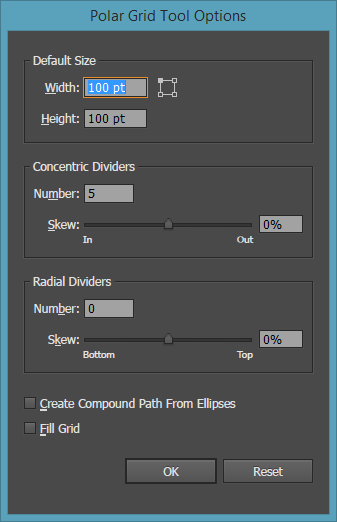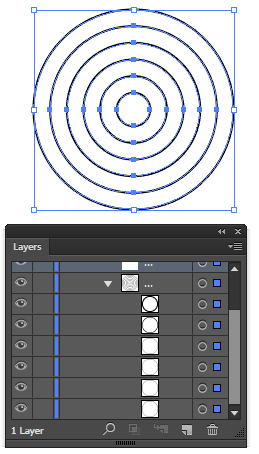Then it’s just a matter of setting the Stroke on the rings to dashed with gaps that fit your liking: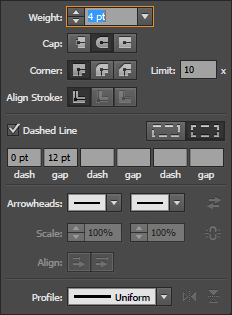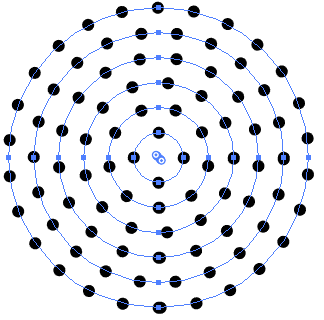You can of course fine tune each row to add more dots if needed, just increase or decrease the individual gap value. With the gap box active, you can use your scroll wheel to quickly change the value. Hold Ctrl / Cmd while scrolling to adjust in finer increments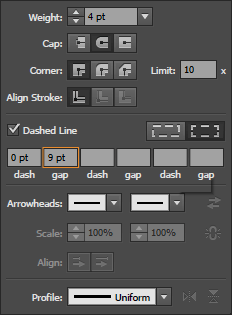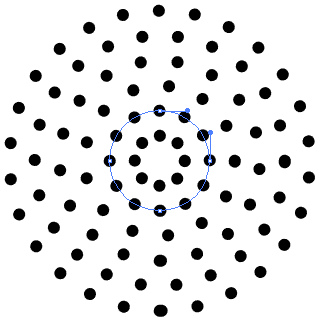An issue with this method is there might be some overlap in some of the dots:Those might need to be manually edited if you need them to be perfect. There should be at most 1 overlap per row.

### Method 4

#### If you do care about the dots lining up…

Illustrator’s distort and transform effect is perfect for this sort of repeating pattern, but in order to get that exact pattern it will require some tweaking. Start with a dotted line (with 11 dots for your example)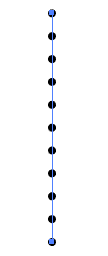Add a Transform Effect via Effect > Distort & Transform > Transform...You’ll notice that the inner rows have too many dots. That’s where the manual tweaking comes in, but this should get you far enough to figure the rest out.

### Method 5

Use Inkscape:

1. Create co-centric guide lines and double click the lines to rotate by equal amount (I used 30 degrees).
2. Create a series of co-centric unfilled circles manually setting the width and height, and moving them to the center.
3. Create a filled circle, and copy paste some number of times.
4. Use the “Rows and Columns” tool to spread them out along a row, with spacing equal
5. Group the circles, and then rotate them. Finally place them so that the center’s line up with the guide intersection.
6. Copy and paste again.And the result (using 22.5 degrees to match the OP’s image):All methods was sourced from stackoverflow.com or stackexchange.com, is licensed under cc by-sa 2.5, cc by-sa 3.0 and cc by-sa 4.0